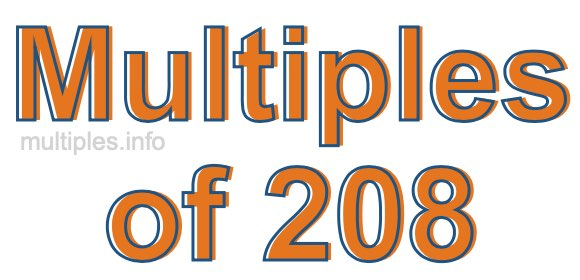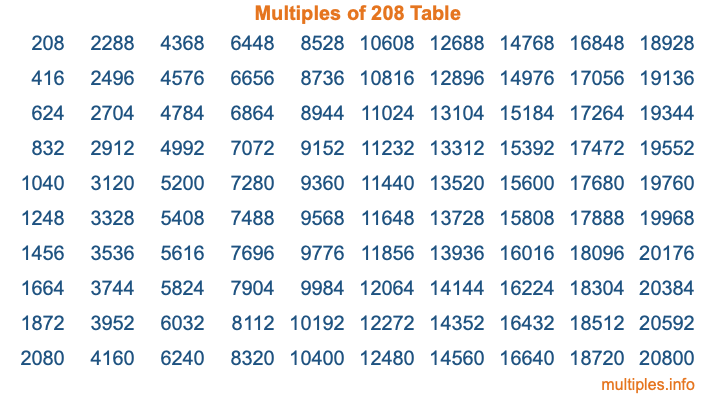Multiples of 208Welcome to the Multiples of 208 page. Here we will first teach you everything you will ever need to know about the multiples of 208, and then give you a study guide summary of everything we taught you to make sure you remember it all. Use this page to look up facts and learn information about the multiples of 208. This page will make you a multiples of two hundred eight expert!

Definition of Multiples of 208
Multiples of 208 are all the numbers that when divided by 208 equal an integer. Each of the multiples of 208 are called a multiple. A multiple of 208 is created by multiplying 208 by an integer.

Therefore, to create a list of multiples of 208, you start with 1 multiplied by 208, then 2 multiplied by 208, then 3 multiplied by 208, and so on for as long as you want. Thus, the list of the first five multiples of 208 is 208, 416, 624, 832, and 1040. To see a larger list of multiples of 208, see the printable image of Multiples of 208 further down on this page. We also have a category where you can choose any nth multiple of 208.

Multiples of 208 Checker
The Multiples of 208 Checker below checks to see if any number of your choice is a multiple of 208. In other words, it checks to see if there is any number (integer) that when multiplied by 208 will equal your number. To do that, we divide your number by 208. If the the quotient is an integer, then your number is a multiple of 208.

Is  a multiple of 208?

Least Common Multiple of 208 and ...
A Least Common Multiple (LCM) is the lowest multiple that two or more numbers have in common. This is also called the smallest common multiple or lowest common multiple and is useful to know when you are adding our subtracting fractions. Enter one or more numbers below (208 is already entered) to find the LCM.

Check out our LCM Calculator if you need more details about the Least Common Multiple or if you need the LCM for different numbers for adding and subtraction fractions.

nth Multiple of 208
As we stated above, 208 is the first multiple of 208, 416 is the second multiple of 208, 624 is the third multiple of 208, and so on. Enter a number below to find the nth multiple of 208.

th multiple of 208

Multiples of 208 vs Factors of 208
208 is a multiple of 208 and a factor of 208, but that is where the similarities end. All postive multiples of 208 are 208 or greater than 208. All positive factors of 208 are 208 or less than 208.

Below is the beginning list of multiples of 208 and the factors of 208 so you can compare:

Multiples of 208: 208, 416, 624, 832, 1040, etc.

Factors of 208: 1, 2, 4, 8, 13, 16, 26, 52, 104, 208

As you can see, the multiples of 208 are all the numbers that you can divide by 208 to get a whole number. The factors of 208, on the other hand, are all the whole numbers that you can multiply by another whole number to get 208.

It's also interesting to note that if a number (x) is a factor of 208, then 208 will also be a multiple of that number (x).

Multiples of 208 vs Divisors of 208
The divisors of 208 are all the integers that 208 can be divided by evenly. Below is a list of the divisors of 208.

Divisors of 208: 1, 2, 4, 8, 13, 16, 26, 52, 104, 208

The interesting thing to note here is that if you take any multiple of 208 and divide it by a divisor of 208, you will see that the quotient is an integer.

Multiples of 208 Table
Below is an image of the first 100 multiples of 208 in a table. The table is in chronological order, column by column. The first column has the first ten multiples of 208, the second column has the next ten multiples of 208, and so on.The Multiples of 208 Table is also referred to as the 208 Times Table or Times Table of 208. You are welcome to print out our table for your studies.

Negative Multiples of 208
Although not often discussed or needed in math, it is worth mentioning that you can make a list of negative multiples of 208 by multiplying 208 by -1, then by -2, then by -3, and so on, to get the following list of negative multiples of 208:

-208, -416, -624, -832, -1040, etc.

Multiples of 208 Summary
Below is a summary of important Multiples of 208 facts that we have discussed on this page. To retain the knowledge on this page, we recommend that you read through the summary and explain to yourself or a study partner why they hold true.

There are an infinite number of multiples of 208.

A multiple of 208 divided by 208 will equal a whole number.

208 divided by a factor of 208 equals a divisor of 208.

The nth multiple of 208 is n times 208.

The largest factor of 208 is equal to the first positive multiple of 208.

208 is a multiple of every factor of 208.

208 is a multiple of 208.

A multiple of 208 divided by a divisor of 208 equals an integer.

208 divided by a divisor of 208 equals a factor of 208.

Any integer times 208 will equal a multiple of 208.

Multiples of a Number
Here you can get the multiples of another number, all with the same attention to detail as we did for multiples of 208 on this page.

Multiples of
Multiples of 209
Did you find our page about multiples of two hundred eight educational? Do you want more knowledge? Check out the multiples of the next number on our list!